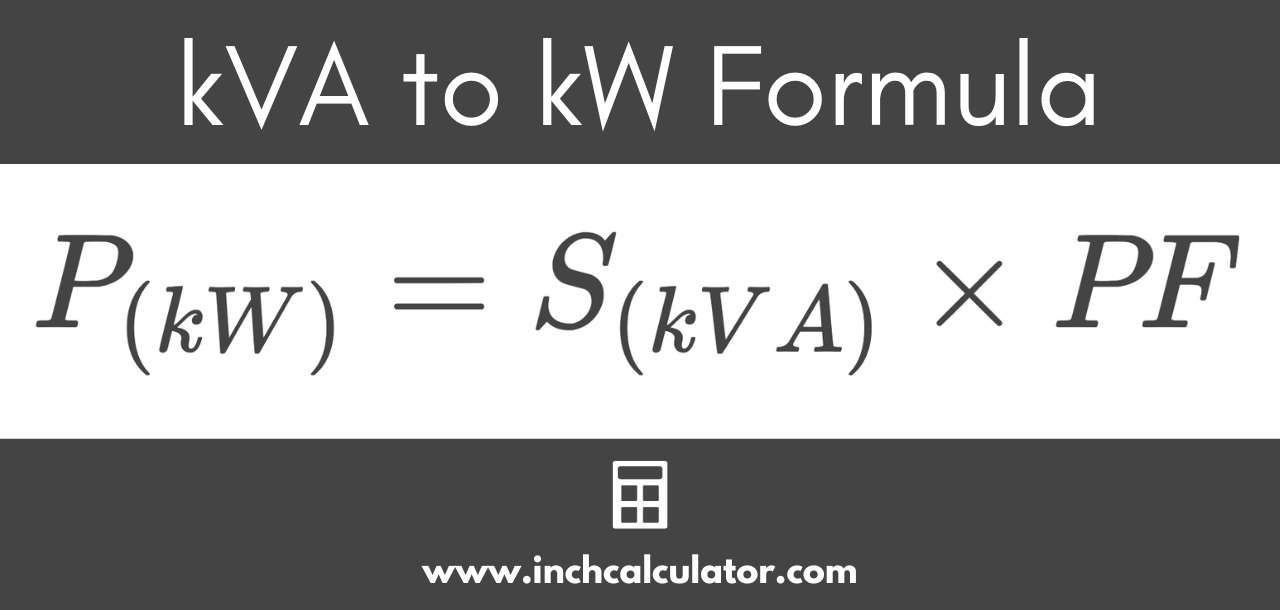# Kilovolt-Amps (kVA) to Kilowatts (kW) Conversion Calculator

Convert kilovolt-amps (kVA) to kilowatts (kW) by entering the apparent power in kVA and the power factor below.

kVA

## Kilowatts Results:

kW
Learn how we calculated this below

## How to Convert kVA to kW

Kilovolt-amps (kVA) and kilowatts (kW) are both measures of power, but they differ slightly. kVA is a measure of the apparent power, while kW is a measure of the real power. These often are different because of equipment power factor.

Power factor is the ratio between apparent power and real power and is a way to represent the amount of power in equipment that’s used to do real work.

The remainder of the power is called reactive power, expressed in Kilovolt-amps-reactive (kVAR), and is stored by the inductive and capacitive elements of the load.

High reactive power can be undesirable as it requires the electrical system to handle a higher overall power load than is actually used, resulting in inefficiencies, wear, and losses to heat.

### Kilovolt-Amps to Kilowatts Formula

To convert from kVA to kW, we must account for the power factor. Use the following formula to convert from kilovolt-amps to kilowatts:

P(kW) = S(kVA) × PF

Thus, the real power P in kilowatts is equal to the apparent power S in kVA times the equipment power factor PF. To convert, simply apply the kVA and power factor to the formula above.For example, let’s find the real power of a piece of equipment with 50 kVA of apparent power and a power factor of 0.8.

P(kW) = 50 kVA × 0.8
P(kW) = 40 kW

So, in this example, 50 kVA can be converted to 40 kW.

## kVA to kW Conversion Chart

The following chart shows kilovolt-amps (kVA) and kilowatt (kW) power conversions for equipment with a power factor of 0.8.

Equivalent kVA and kW ratings given a power factor of 0.8.
kVA kW
6.3 kVA 5 kW
9.4 kVA 7.5 kW
12.5 kVA 10 kW
18.7 kVA 15 kW
25 kVA 20 kW
31.3 kVA 25 kW
37.5 kVA 30 kW
50 kVA 40 kW
62.5 kVA 50 kW
75 kVA 60 kW
93.8 kVA 75 kW
100 kVA 80 kW
125 kVA 100 kW
156 kVA 125 kW
187 kVA 150 kW
219 kVA 175 kW
250 kVA 200 kW
312 kVA 250 kW
375 kVA 300 kW
438 kVA 350 kW
500 kVA 400 kW
625 kVA 500 kW
750 kVA 600 kW
875 kVA 700 kW
1,000 kVA 800 kW
1,125 kVA 900 kW
1,250 kVA 1,000 kW
1,563 kVA 1,250 kW
1,875 kVA 1,500 kW
2,188 kVA 1,750 kW
2,500 kVA 2,000 kW
2,812 kVA 2,250 kW

Do you want to convert kW to kVA?